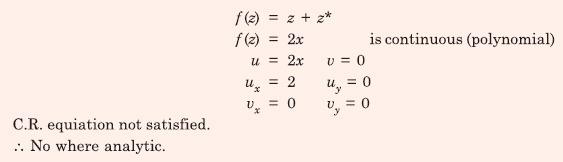# Signals and Systems - Online Test

Q1. For linear time invariant systems, that are Bounded Input Bounded stable, which one of the following statement is TRUE?
Explaination / Solution:
No Explaination.

Q2. Consider a signal defined by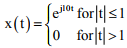Its Fourier Transform is
Explaination / Solution: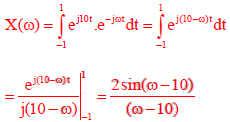Q3. The following discrete-time equations result from the numerical integration of the differential equations of an un-damped simple harmonic oscillator with state variables x and y. The integration time step is h.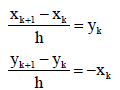For this discrete-time system, which one of the following statements is TRUE?
Explaination / Solution:
No Explaination.

Q4. A function y(t), such that y(0) = 1 and y(1) = 3e-1, is a solution of the differential equationThen y(2) is
Explaination / Solution: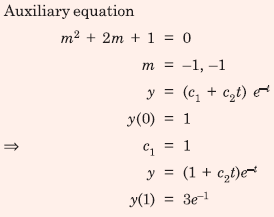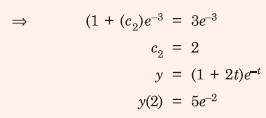Q5. The value of the integralover the contour |z| = 1, taken in the anti-clockwise direction, would be
Explaination / Solution: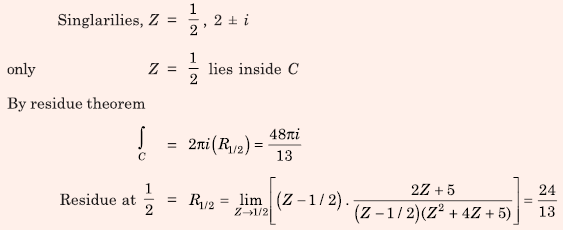Q6. Consider a continuous-time system with input x(t) and output y(t) given by y(t) = x(t) cos(t) This system is
Explaination / Solution:Q7. Suppose x1(t) and x2(t) have the Fourier transforms as shown below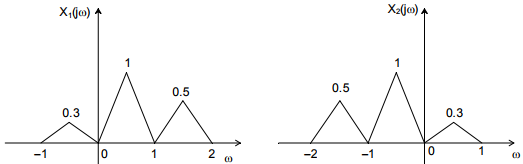Which one of the following statements is TRUE?
Explaination / Solution: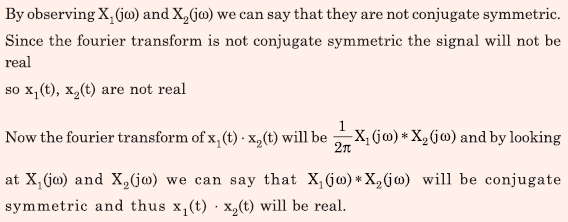Q8. The output of a continuous-time, linear time-invariant system is denoted by T{x(t)} where x(t) is the input signal. A signal z(t) is called eigen-signal of the system T , when T{z(t)} = γz(t), where γ is a complex number, in general, and is called an eigenvalue of T. Suppose the impulse response of the system T is real and even. Which of the following statements is TRUE?
Explaination / Solution:Q9. Consider a causal LTI system characterized by differential equation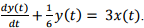The response of the system to the inputwhere u(t) denotes the unit step function, is
Explaination / Solution: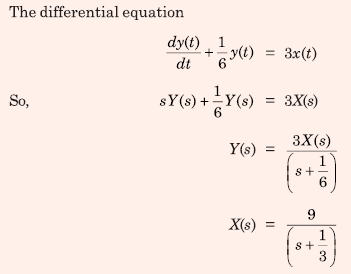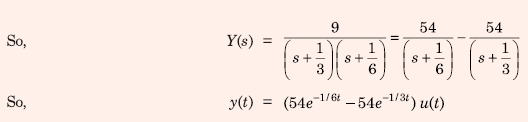Q10. Consider the function ݂f(z) = z + z* where z is a complex variable and z* denotes its complex conjugate. Which one of the following is TRUE?
Explaination / Solution: